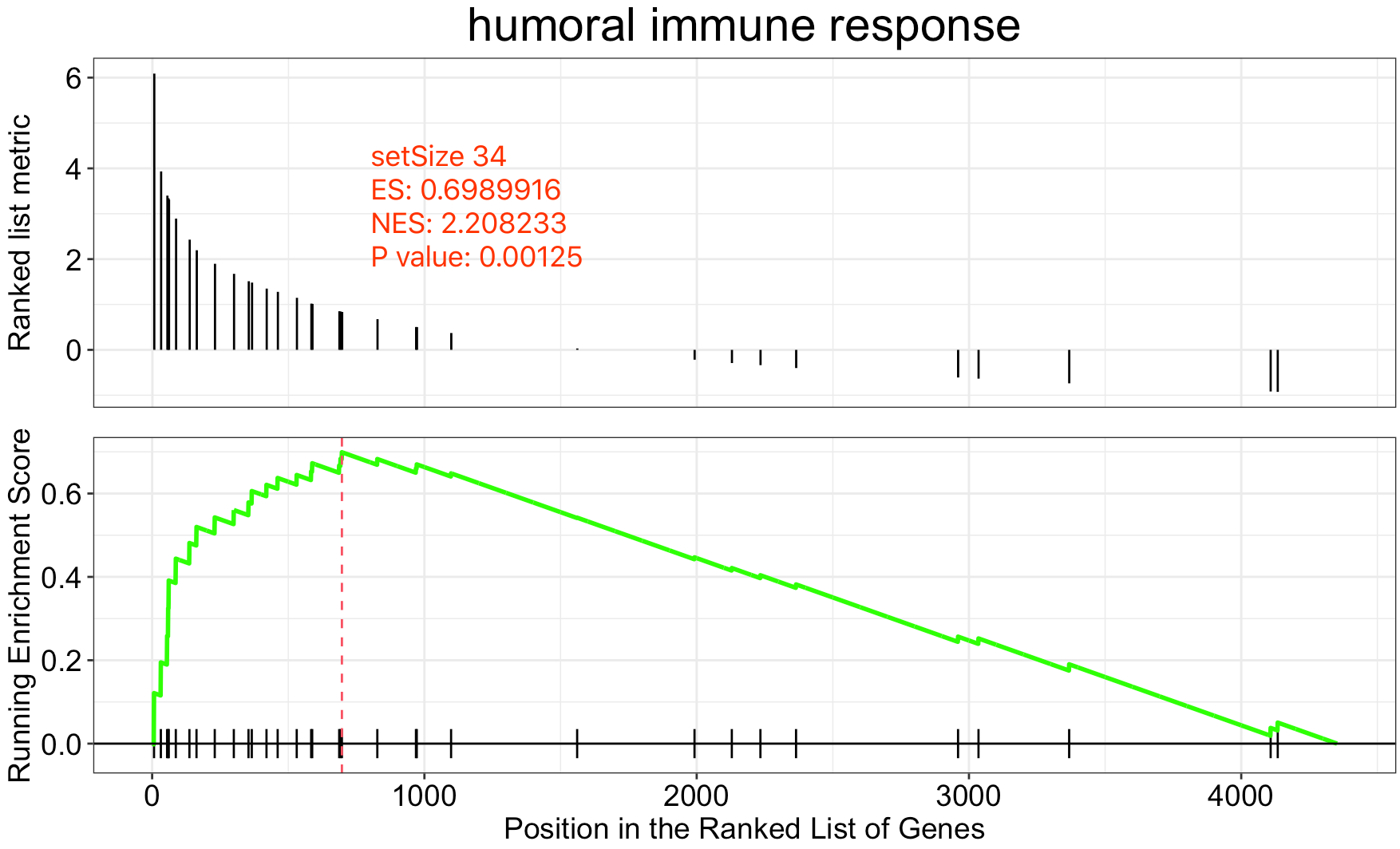做GSEA分析你的基因到底该如何排序• signal-to-noise ratio (S2N)
• ratio of average expression from two classes (Ratio)
• T-test statistic (T-test)
• the Pearson correlation coefficient for quantitative studies

12种排序方法

``````The absolute value of Moderated Welch Test has the best overall sensitivity and Minimum Significant Difference has the best overall specificity of gene set analysis.
``````

T-test
MWT
\ MWT\
MSD
S2N
\ S2N\
Difference
Ratio
log2(Ratio)
FCROS
SoR
BWS
ReliefF
ReliefF ranked

如果是DESeq2的差异分析结果

``````x\$fcsign <- sign(x\$log2.fold_change)
x\$logP=-log10(x\$p_value)
x\$metric= x\$logP/x\$fcsign
``````